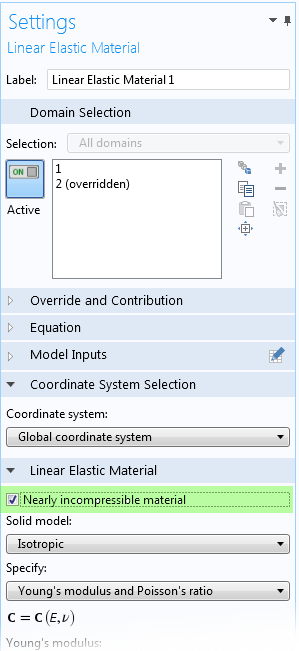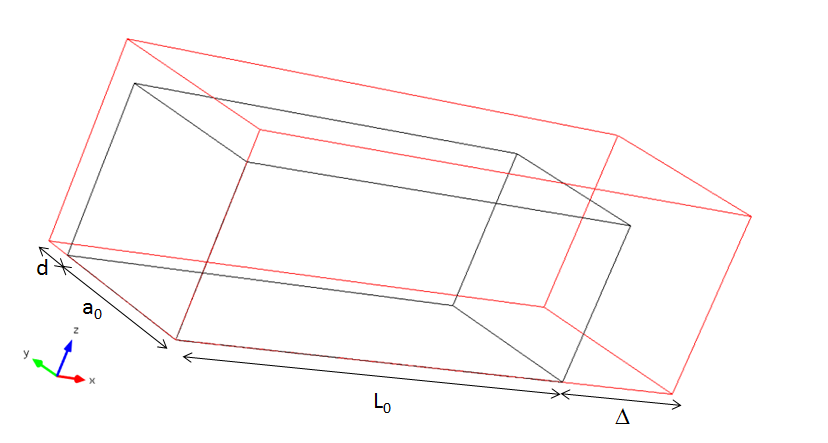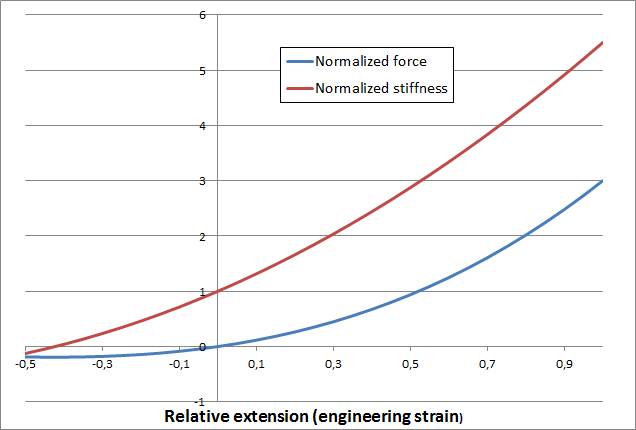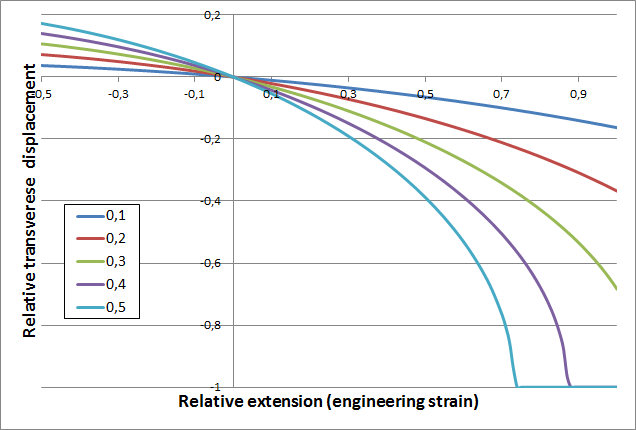# 模拟线弹性材料能有多难？

2015年 6月 29日

### 各向同性线弹性

#### 杨氏模量、剪切模量和泊松比

G = \frac{E}{2(1+\nu)}

\frac{1}{3} < \frac{G}{E} < \infty

\nu 接近 0.5 时，材料变得不可压缩，此类材料会给分析带来特定问题，我们将在下文说明。

#### 体积模量

K = \frac{E}{3(1-2\nu)}

\nu= 1/3 时，体积模量与杨氏模量的值相等，但是对于不可压缩材料（\nu \to0.5），K 趋近无穷。

#### 拉梅常数

\boldsymbol \sigma=2\mu \boldsymbol \varepsilon +\lambda \; \mathrm{trace}(\boldsymbol{\varepsilon}) \mathbf I

\lambda = \frac{E \nu}{(1+\nu)(1-2\nu)}

### 线弹性材料的不可压缩性

p = f(\Delta V)

\Delta V = 0

• 模型过于生硬。
• 应力呈棋盘式分布。
• 方程的病态导致求解器发生错误或发出警告。### 正交各向异性和各向异性

\boldsymbol \sigma= \mathbf D \boldsymbol \varepsilon

\mathsf{C} =
\begin{bmatrix}
\tfrac{1}{E_{\rm X}} & -\tfrac{\nu_{\rm YX}}{E_{\rm Y}} & -\tfrac{\nu_{\rm ZX}}{E_{\rm Z}} & 0 & 0 & 0 \\
-\tfrac{\nu_{\rm XY}}{E_{\rm X}} & \tfrac{1}{E_{\rm Y}} & -\tfrac{\nu_{\rm ZY}}{E_{\rm Z}} & 0 & 0 & 0 \\
-\tfrac{\nu_{\rm XZ}}{E_{\rm X}} & -\tfrac{\nu_{\rm YZ}}{E_{\rm Y}} & \tfrac{1}{E_{\rm Z}} & 0 & 0 & 0 \\
0 & 0 & 0 & \tfrac{1}{G_{\rm YZ}} & 0 & 0 \\
0 & 0 & 0 & 0 & \tfrac{1}{G_{\rm ZX}} & 0 \\
0 & 0 & 0 & 0 & 0 & \tfrac{1}{G_{\rm XY}} \\
\end{bmatrix}

\tfrac{\nu_{\rm YX}}{E_Y} = \tfrac{\nu_{\rm XY }}{E_X}

1. 本构矩阵 \mathbf D 必须是正定的。
1. 对于各向异性材料，唯一的选择是检查是否所有特征值都为正。
2. 对于正交各向异性材料，适用条件为：全部六个弹性模量皆为正，且 \nu_{\rm XY}\nu_{\rm YX}+\nu_{\rm YZ}\nu_{\rm ZY}+\nu_{\rm ZX}\nu_{\rm XZ}+\nu_{\rm YX}\nu_{\rm ZY}\nu_{\rm XZ}<1
2. 如果材料的压缩率低，则必须使用混合方程。
1. 我们可以估计等效体积模量和剪切模量的值。
2. 在不确定的情况下，为了避免可能出现的误差，最好在混合方程中引入额外自由度。

### 几何非线性F = \sigma_x A = \sigma_x A_0 (1+\eta)^2

\mathbf \sigma = J^{-1} \mathbf F \mathbf S \mathbf F^T

\sigma_x = \frac{F_{xX}}{F_{yY}F_{zZ}}S_X= \frac{(1+\xi)}{(1+\eta)^2}S_X

F = S_x A_0 (1+\xi) = E A_0 (1+\xi)\varepsilon_X

\varepsilon_X = \frac{\partial u}{\partial X} + \frac{1}{2}(\frac{\partial u}{\partial X})^2 = \xi+\frac{1}{2}\xi^2

F = E A_0 (1+\xi)(\xi + \frac{1}{2}\xi^2)=E A_0 (\xi+\frac{3}{2}\xi^2+\frac{1}{2}\xi^3)• 通常，在引入更复杂的材料模型之前，您可能想快速检查一遍“数量级”。
• 模型中存在奇异点，并且致使某一点上产生了极高的应变。
• 接触问题中的研究总是围绕几何非线性问题。
• 高压缩应变通常在分析过程中出现于某一时刻的局部接触区域。

\varepsilon_Y = -\nu \varepsilon_X

\frac{\partial v}{\partial Y} + \frac{1}{2}(\frac{\partial v}{\partial Y})^2 = -\nu (\frac{\partial u}{\partial X} + \frac{1}{2}(\frac{\partial u}{\partial X})^2)

\eta = \sqrt{1-\nu(\xi^2+2\xi)}-1St. Venant 材料受到单轴拉伸作用时，其横向位移随轴向位移而变化。图中显示了五个不同的泊松比的值。